﻿ Lamb波在抛物线型声黑洞薄板结构中的传播特性

# Lamb波在抛物线型声黑洞薄板结构中的传播特性Study the Propagation Characteristics of Lamb Waves on Parabolic Acoustic Black Hole Thin Plate Structures

Abstract: In this paper, the propagation characteristics of Lamb waves on thin plate structure with parabolic acoustic black hole are studied by using laser ultrasonic technology. Based on the thermoelastic mechanism, a three-dimensional finite element model of laser excitation of Lamb wave in thin plate structure with parabolic acoustic black hole is established. Then, the acoustic wave propagates outward as the excitation region is the centre; the wave is parallel to the laser line source; and its propagation direction is perpendicular to the laser line source. When the sound wave encounters the acoustic black hole, it will propagate along the thickness decreasing direction. Meanwhile, the acoustic beam width decreases, and the amplitude gradually increases. Finally, the acoustic amplitude at the lowest point of the acoustic black hole increases gradually, which present the sound wave focusing phenomenon. The results of this paper can be used to detect and evaluate the thin plate structure with acoustic black hole.

1. 引言

Lamb波是平板按自由边界条件解波动方程时得到的一种特殊的波动解 ，被广泛应用于两个平行表面结构中的无损检测。利用其能量集中在薄板结构的上、下两个平面内，传播过程中衰减，可以实现非接触式激发，对于大规模薄板结构的无损检测具有极其重要的意义 。

2. 理论研究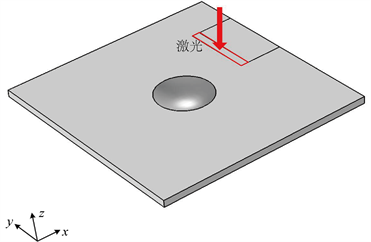Figure 1. Schematic diagram of laser irradiation two-dimensional parabolic acoustic black hole structures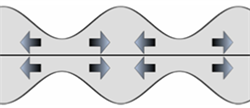(a) 对称模态的Lamb波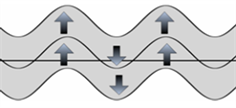(b) 反对称模态的Lamb波

Figure 2. Schematic diagram of symmetry and antisymmetry Lamb wave mode

$-k\frac{\partial T\left(x,y,z,t\right)}{\partial n}={I}_{0}A\left(T\right)f\left(x\right)g\left(t\right)$ (1)

$g\left(t\right)=\frac{8{t}^{3}}{{t}_{0}^{4}}\mathrm{exp}\left(\frac{-2{t}^{2}}{{t}_{0}^{2}}\right)$ (2)

$f\left(x\right)=\frac{1}{\sqrt{2\pi }}\frac{2}{{R}_{G}}\mathrm{exp}\left(\frac{-2{\left(x-{x}_{0}\right)}^{2}}{{R}_{G}^{2}}\right)$ (3)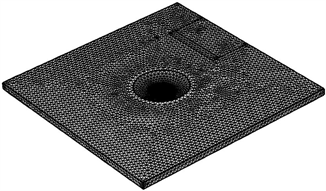Figure 3. The meshing of three-dimensional parabolic acoustic black hole structureTable 1. Parameters of aluminum

3. 结果与讨论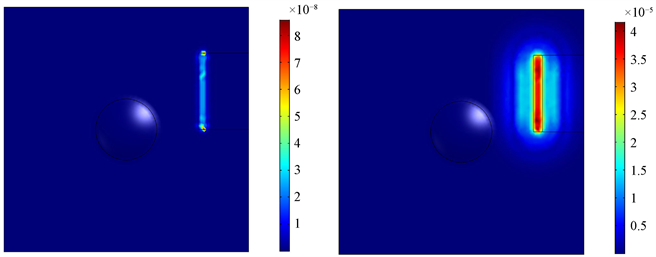(a) t = 0 μs (b) t = 0.5 μs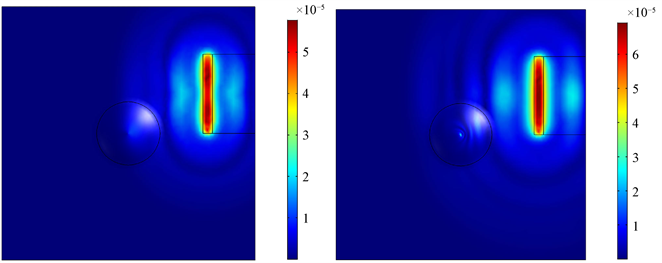(c) t = 1 μs (d) t = 1.5 μs

Figure 4. The displacement field of Lamb wave at 0 μs, 0.5 μs, 1 μs, 1.5 μs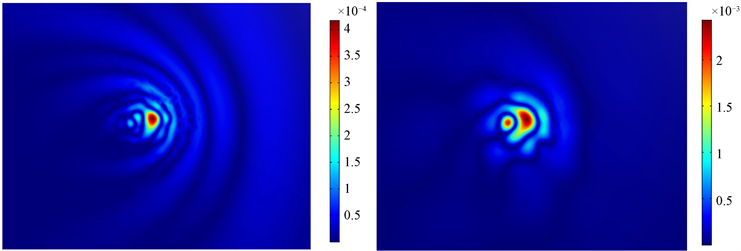(a) t = 2 μs (b) t = 3 μs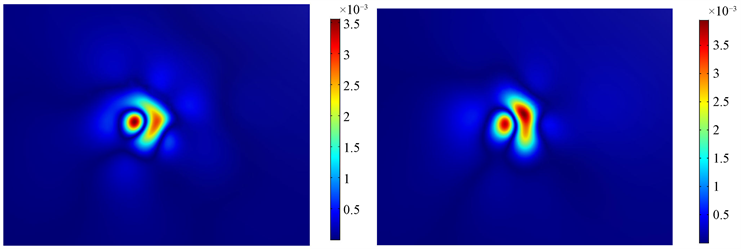(c) t = 4 μs (d) t = 5 μs

Figure 5. The displacement field of black hole at 2 μs, 3 μs, 4 μs, 5 μs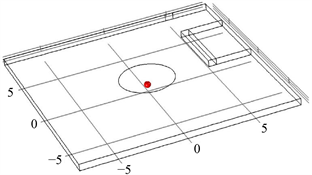Figure 6. Schematic diagram of the center of black hole at (0 mm, 0 mm, 0 mm)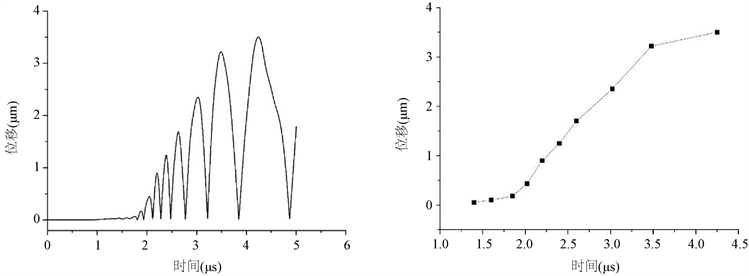(a) (b)

Figure 7. (a) The displacement-time diagram and (b) the change of the peak displacement of the black hole center

4. 总结

 Lamb, H. (1917) On Waves in an Elastic Plate. Proceedings of the Royal Society of London, Containing Paper of a Mathematical and Physical Character, 93, 114-128.
https://doi.org/10.1098/rspa.1917.0008

 Rose J L, 何存富, 吴斌, 王秀彦. 固体中的超声波[M]. 北京: 科学出版社, 2004.

 Johnson, M.A., Berthelot, Y.H., Brodeur, P. and Gerhardstein, J. (1995) Investigation of Lamb Waves in Copy Paper Using Laser Ultrasonics. The Journal of the Acoustical Society of America, 98, 2916.
https://doi.org/10.1121/1.414211

 Wu, T.-Y. and Ume, I.C. (2011) Fundamental Study of Laser Generation of Narrowband Lamb Waves Using Superimposed Line Sources Technique. NDT & E International, 44, 315-323.
https://doi.org/10.1016/j.ndteint.2011.01.006

 孙宏祥, 许伯强. 激光激发Lamb波的有限元时域和频域数值分析[J]. 中国激光, 2010, 37(2): 537-542.

 许伯强, 陈丽娟, 徐桂东, 徐晨光, 骆英. 各向异性板中激光激发Lamb波的数值模拟[J]. 中国激光, 2012, 39(3): 94-99.

 赵振宁, 吴迪, 张博南, 吴军芳, 滕永平. 薄板中超声导波传播模态信号分析方法[J]. 无损检测, 2017, 39(1):1 0-15.

 Krylov, V.V. (2002) Acoustic ‘black holes’ for Flexural Waves and Their Potential Applications. Institute of Acoustics Spring Conference, Salford, 5-27 March 2002, 25-27.

 Yan, S.L., Lomonosov, A.M. and Shen, Z.H. (2016) Numerical and Experimental Study of Lamb Wave Propagation in a Two-Dimensional Acoustic Black Hole. Journal of Applied Physics, 119, Article ID: 214902.
https://doi.org/10.1063/1.4953221

 罗静. 基于激光超声技术的一维声学黑洞结构研究[D]: [硕士学位论文]. 南京: 南京航空航天大学, 2017.

 黄薇, 季宏丽, 裘进浩, 成利. 二维声学黑洞对弯曲波的能量聚集效应[J]. 振动与冲击, 2017, 36(9): 51-57.

 Jia, J., Shen, Z., Han, Q. and Jing, X.P. (2017) Design of Wedge Structure with Non-Dispersive Wedge Wave Propagation. Applied Optics, 56, 8564-8569.
https://doi.org/10.1364/AO.56.008564

Top